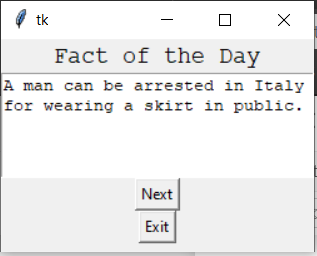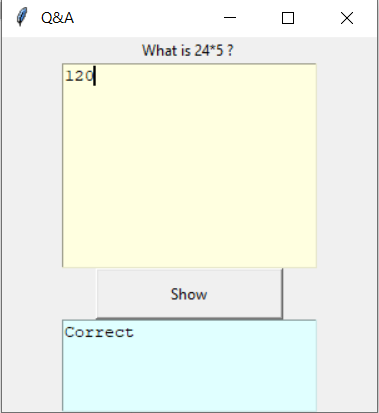# Python Tkinter – Text Widget

• Difficulty Level : Easy
• Last Updated : 24 Jan, 2022

Tkinter is a GUI toolkit used in python to make user-friendly GUIs.Tkinter is the most commonly used and the most basic GUI framework available in python. Tkinter uses an object-oriented approach to make GUIs.
Note: For more information, refer to Python GUI – tkinter

## Text Widget

Text Widget is used where a user wants to insert multiline text fields. This widget can be used for a variety of applications where the multiline text is required such as messaging, sending information or displaying information and many other tasks. We can insert media files such as images and links also in the Textwidget.
Syntax:

`T = Text(root, bg, fg, bd, height, width, font, ..)`

Optional parameters

• root – root window.

• bg – background colour

• fg – foreground colour

• bd – border of widget.

• height – height of the widget.

• width – width of the widget.

• font – Font type of the text.

• cursor – The type of the cursor to be used.

• insetofftime – The time in milliseconds for which the cursor blink is off.

• insertontime – the time in milliseconds for which the cursor blink is on.

• state – defines if the widget will be responsive to mouse or keyboards movements.

• highlightthickness – defines the thickness of the focus highlight.

• insertionwidth – defines the width of insertion character.

• relief – type of the border which can be SUNKEN, RAISED, GROOVE and RIDGE.

• yscrollcommand – to make the widget vertically scrollable.

• xscrollcommand – to make the widget horizontally scrollable.

Some Common methods

• index(index) – To get the specified index.

• insert(index) – To insert a string at a specified index.

• see(index) – Checks if a string is visible or not at a given index.

• get(startindex, endindex) – to get characters within a given range.

• delete(startindex, endindex) – deletes characters within specified range.

Tag handling methods

• tag_delete(tagname) – To delete a given tag.

• tag_add(tagname, startindex, endindex) – to tag the string in the specified range

• tag_remove(tagname, startindex, endindex) – to remove a tag from specified range

Mark handling methods

• mark_names() – to get all the marks in the given range.

• index(mark) – to get index of a mark.

• mark_gravity() – to get the gravity of a given mark.

Example 1:

## Python3

 `import` `tkinter as tk`     `root ``=` `Tk()`   `# specify size of window.` `root.geometry(``"250x170"``)`   `# Create text widget and specify size.` `T ``=` `Text(root, height ``=` `5``, width ``=` `52``)`   `# Create label` `l ``=` `Label(root, text ``=` `"Fact of the Day"``)` `l.config(font ``=``(``"Courier"``, ``14``))`   `Fact ``=` `"""A man can be arrested in` `Italy for wearing a skirt in public."""`   `# Create button for next text.` `b1 ``=` `Button(root, text ``=` `"Next"``, )`   `# Create an Exit button.` `b2 ``=` `Button(root, text ``=` `"Exit"``,` `            ``command ``=` `root.destroy) `   `l.pack()` `T.pack()` `b1.pack()` `b2.pack()`   `# Insert The Fact.` `T.insert(tk.END, Fact)`   `tk.mainloop()`

OutputExample 2: Saving Text and performing operations

## Python3

 `from` `tkinter ``import` `*`   `root ``=` `Tk()` `root.geometry(``"300x300"``)` `root.title(``" Q&A "``)`   `def` `Take_input():` `    ``INPUT` `=` `inputtxt.get(``"1.0"``, ``"end-1c"``)` `    ``print``(``INPUT``)` `    ``if``(``INPUT` `=``=` `"120"``):` `        ``Output.insert(END, ``'Correct'``)` `    ``else``:` `        ``Output.insert(END, ``"Wrong answer"``)` `    `  `l ``=` `Label(text ``=` `"What is 24 * 5 ? "``)` `inputtxt ``=` `Text(root, height ``=` `10``,` `                ``width ``=` `25``,` `                ``bg ``=` `"light yellow"``)`   `Output ``=` `Text(root, height ``=` `5``, ` `              ``width ``=` `25``, ` `              ``bg ``=` `"light cyan"``)`   `Display ``=` `Button(root, height ``=` `2``,` `                 ``width ``=` `20``, ` `                 ``text ``=``"Show"``,` `                 ``command ``=` `lambda``:Take_input())`   `l.pack()` `inputtxt.pack()` `Display.pack()` `Output.pack()`   `mainloop()`

OutputMy Personal Notes arrow_drop_up
Recommended Articles
Page :